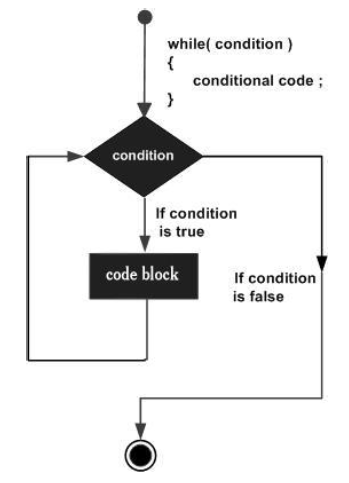# TypeScript - While Loop

The while loop executes the instructions each time the condition specified evaluates to true. In other words, the loop evaluates the condition before the block of code is executed.

## Syntax

```while(condition) {
// statements if the condition is true
}
```

## Flow Diagram## Example: While loop

```var num:number = 5;
var factorial:number = 1;

while(num >=1) {
factorial = factorial * num;
num--;
}
console.log("The factorial  is "+factorial);
```

The above code snippet uses a while loop to calculate the factorial of the value in the variable num.

On compiling, it will generate the following JavaScript code −

```//Generated by typescript 1.8.10
var num = 5;
var factorial = 1;
while (num >= 1) {
factorial = factorial * num;
num--;
}
console.log("The factorial  is " + factorial);
```

It produces the following output −

```The factorial is 120
```
typescript_loops.htm